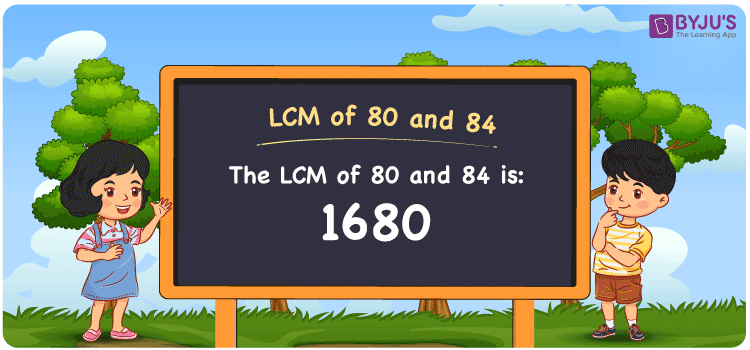# LCM of 80 and 84

LCM of 80 and 84 is 1680. The smallest number among all common multiples of 80 and 84 is the LCM of 80 and 84. (80, 160, 240, 320, etc.) and (84, 168, 252, 336, 420, 504, etc.) are the first few multiples of 80 and 84, respectively. To find the LCM of 80 and 84, there are three typical methods: prime factorization, division method, and listing multiples. The LCM of any two integers in mathematics is the value that is evenly divisible by the two values.

Also read: Least common multiple

## What is LCM of 80 and 84?

The answer to this question is 1680. The LCM of 80 and 84 using various methods is shown in this article for your reference. The LCM of two non-zero integers, 80 and 84, is the smallest positive integer 1680 which is divisible by both 80 and 84 with no remainder.## How to Find LCM of 80 and 84?

LCM of 80 and 84 can be found using three methods:

• Prime Factorisation
• Division method
• Listing the multiples

### LCM of 80 and 84 Using Prime Factorisation Method

The prime factorisation of 80 and 84, respectively, is given by:

80 = (2 × 2 × 2 × 2 × 5) = 24 × 51 and

84 = (2 × 2 × 3 × 7) = 22 × 31 × 71

LCM (80, 84) = 1680

### LCM of 80 and 84 Using Division Method

We’ll divide the numbers (80, 84) by their prime factors to get the LCM of 80 and 84 using the division method (preferably common). The LCM of 80 and 84 is calculated by multiplying these divisors.

 2 80 84 2 40 42 2 20 21 2 10 21 3 5 21 5 5 7 7 1 7 x 1 1

No further division can be done.

Hence, LCM (80, 84) = 1680

### LCM of 80 and 84 Using Listing the Multiples

To calculate the LCM of 80 and 84 by listing out the common multiples, list the multiples as shown below

 Multiples of 80 Multiples of 84 80 84 160 168 240 252 ….. …… 1680 1680

The smallest common multiple of 80 and 84 is 1680.

Therefore LCM (80, 84) = 1680

## Video Lesson on Applications of LCM## LCM of 80 and 84 Solved Example

The product of two numbers is 6720. If their GCD is 4, what is their LCM?

Solution:

Given: GCD = 4

product of numbers = 6720

∵ LCM × GCD = product of numbers

⇒ LCM = Product/GCD = 6720/4

Therefore, the LCM is 1680.

The probable combination for the given case is LCM(80, 84) = 1680.

## Frequently Asked Questions on LCM of 80 and 84

### What is the LCM of 80 and 84?

The LCM of 80 and 84 is 1680. To find the LCM (least common multiple) of 80 and 84, we need to find the multiples of 80 and 84 (multiples of 80 = 80, 160, 240, 320 . . . . 1680; multiples of 84 = 84, 168, 252, 336 . . . . 1680) and choose the smallest multiple that is exactly divisible by 80 and 84, i.e., 1680.

### List the methods used to find the LCM of 80 and 84.

The methods used to find the LCM of 80 and 84 are Prime Factorization Method, Division Method and Listing multiples.

### What is the Least Perfect Square Divisible by 80 and 84?

The least number divisible by 80 and 84 = LCM(80, 84)
LCM of 80 and 84 = 2 × 2 × 2 × 2 × 3 × 5 × 7 [Incomplete pair(s): 3, 5, 7] ⇒ Least perfect square divisible by each 80 and 84 = LCM(80, 84) × 3 × 5 × 7 = 176400 [Square root of 176400 = √176400 = ±420] Therefore, 176400 is the required number.

### If the LCM of 84 and 80 is 1680, Find its GCF.

LCM(84, 80) × GCF(84, 80) = 84 × 80
Since the LCM of 84 and 80 = 1680
⇒ 1680 × GCF(84, 80) = 6720
Therefore, the GCF (greatest common factor) = 6720/1680 = 4.

### Which of the following is the LCM of 80 and 84? 28, 45, 15, 1680

The value of LCM of 80, 84 is the smallest common multiple of 80 and 84. The number satisfying the given condition is 1680.